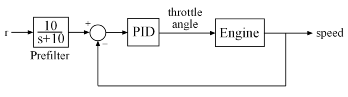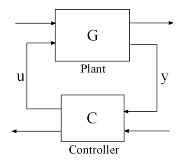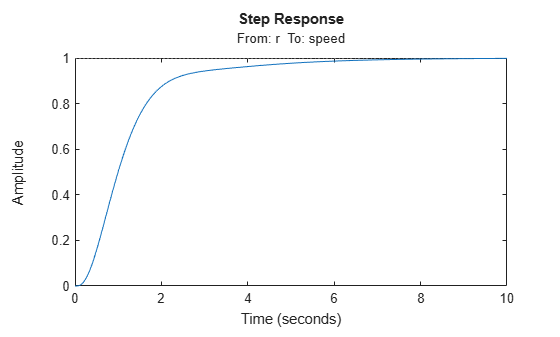# Tune Feedback Loops using `looptune`

This example shows the basic workflow of tuning feedback loops with the `looptune` command. `looptune` is similar to `systune` and meant to facilitate loop shaping design by automatically generating the tuning requirements.

### Engine Speed Control

This example uses a simple engine speed control application as shown in the following figure. The control system consists of a single PID loop and the PID controller gains must be tuned to adequately respond to step changes in the desired speed. Specifically, you want the response to settle in less than 5 seconds with little or no overshoot.Use the following fourth-order model of the engine dynamics.

```load rctExamples Engine bode(Engine) grid```### Specify Tunable Elements

You need to tune the four PID gains to achieve the desired performance. Use a `tunablePID` object to parameterize the PID controller.

`PID0 = tunablePID('SpeedController','pid')`
```PID0 = Tunable continuous-time PID controller "SpeedController" with formula: 1 s Kp + Ki * --- + Kd * -------- s Tf*s+1 and tunable parameters Kp, Ki, Kd, Tf. Type "pid(PID0)" to see the current value and "get(PID0)" to see all properties. ```

### Build Tunable Model of Feedback Loop

`looptune` tunes the generic SISO or MIMO feedback loop shown in the following figure. This feedback loop models the interaction between the plant and the controller. Note that this is a positive feedback interconnection.For the speed control loop, the plant $G$ is the engine model and the controller $C$ consists of a PID controller and prefilter $F$.To use `looptune`, create models for $G$ and $C$. Assign names to the inputs and outputs of each model to specify the feedback paths between plant and controller. Note that the controller $C$ has two inputs: the speed reference `r` and the speed measurement `speed`.

```F = tf(10,[1 10]); % prefilter G = Engine; G.InputName = 'throttle'; G.OutputName = 'speed'; C0 = PID0 * [F , -1]; C0.InputName = {'r','speed'}; C0.OutputName = 'throttle';```

Here, `C0` is a generalized state-space model (`genss`) that depends on the tunable PID block `PID0`.

### Tune Controller Parameters

You can now use `looptune` to tune the PID gains subject to a simple control bandwidth requirement. To achieve the 5-second settling time, the gain crossover frequency of the open-loop response should be approximately 1 rad/s. Given this basic requirement, `looptune` automatically shapes the open-loop response to provide integral action, high-frequency roll-off, and adequate stability margins. Note that you could specify additional requirements to further constrain the design. For an example, see Decoupling Controller for a Distillation Column.

```wc = 1; % target gain crossover frequency [~,C,~,Info] = looptune(G,C0,wc);```
```Final: Peak gain = 0.892, Iterations = 7 Achieved target gain value TargetGain=1. ```

The final value is less than 1, indicating that the desired bandwidth was achieved with adequate roll-off and stability margins. `looptune` returns the tuned controller `C`. Use `getBlockValue` to retrieve the tuned value of the PID block.

`PIDT = getBlockValue(C,'SpeedController')`
```PIDT = 1 s Kp + Ki * --- + Kd * -------- s Tf*s+1 with Kp = 0.000958, Ki = 0.00288, Kd = 0.00014, Tf = 0.845 Name: SpeedController Continuous-time PIDF controller in parallel form. ```

### Validate Results

Use `loopview` to validate the design and visualize the loop shaping requirements implicitly enforced by `looptune`.

```clf loopview(G,C,Info)```Next, plot the closed-loop response to a step command in engine speed. The tuned response satisfies the requirements.

```T = connect(G,C,'r','speed'); % closed-loop transfer function from r to speed clf step(T)```